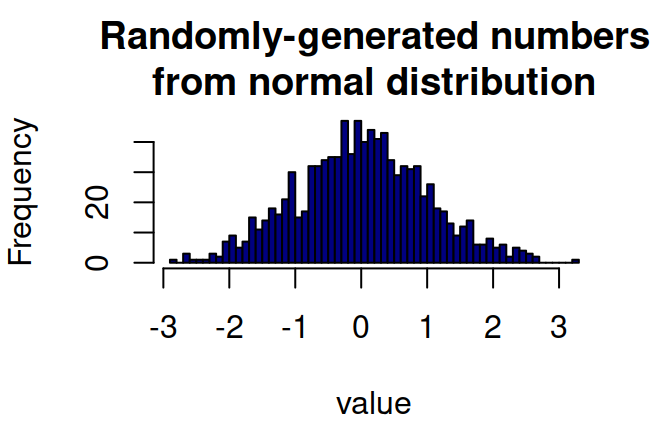This is an accompanying vignette for the package isotree presenting a short introduction to the Isolation Forest family of algorithms as implemented in said package. For more information about it, see the GitHub page.

# Isolation Forests

## Overview

Isolation Forest is an unsupervised decision-tree-based algorithm originally developed for outlier detection in tabular data, which consists in splitting sub-samples of the data according to some attribute/feature/column at random. The idea is that, the rarer the observation, the more likely it is that a random split on some feature would put outliers alone in one branch, and the fewer splits it will take to isolate (create a partition in which only one point is present) an outlier observation like this.

The intuition behind it is very simple: if there is an outlier in the data and we pick a column at random in which the value for the outlier point is different from the rest of the observations, and then we select an arbitrary threshold uniformly at random within the range of that column and divide all the points into two groups according to whether they are higher or lower than the randomly-chosen threshold for that column, then there is a higher chance that the outlier point would end up in the smaller partition than in the larger partition.

Of course, outliers are typically not defined by just having one extreme value in one column, and a good outlier detection method needs to look at the relationships between different variables and their combinations. One potential way to do this is by building a so-called “isolation tree”, which consists of repeating the randomized splitting process described above recursively (that is, we divide the points into two groups, then repeat the process in each of the two groups that are obtained, and continue repeating it on the new groups until no further split is possible or until meeting some other criteria).

Under this scheme, one can deduce that, the more common a point is, the more splits it will take to leave the point alone or in a smaller group compared to uncommon points - as such, one can think of the “isolation depth” (number of partitions that it takes to isolate a point, hence the name of the algorithm) in the isolation trees as a metric by which to measure the inlierness or outlierness of a point.

A single isolation tree has a lot of expected variability in the isolation depths that it will give to each observation, thus an ensemble of many such trees - an “isolation forest” - may be used instead for better results, with the final score obtained by averaging the results (the isolation depths) from many such trees.

There are many potential ways of improving upon the logic behind the procedure (for example, extrapolating an isolation depth after reaching a certain limit) and the resulting score can be standardized for easier usage, among many others - see the references for more details about the methodology.

## Why choose isolation forests over the alternatives

Compared to other outlier/anomaly detection methods such as “local outlier factor” or “one-class support vector machines”, isolation forests have advantages in that they are:

• Robust to the presence of outliers in training data.
• Robust to multi-modal distributions.
• Insensitive to the scales of variables.
• Much faster to fit.
• Invariant to the choice of distance metric (since it doesn’t use a distance metric in the first place).

Additionally, since they produce a standardized outlier metric for every point, such models can be used for example to generate additional features for regression or classification models or as a proxy for distribution density, for which not all outlier detection methods are equally suitable (see the rest of the vignette for other potential uses of isolation forests).

## An example in 1D

As a simple proof-of-concept test, one can think of producing random numbers from a normal distribution and seeing what kinds of isolation depths would isolation trees assigning to them:

``````set.seed(123)
random_numbers <- matrix(rnorm(1000))
par(oma = c(0,0,0,0), mar = c(4,4,3,2))
hist(random_numbers, breaks=50, col="navy",
main="Randomly-generated numbers\nfrom normal distribution",
xlab="value")````````````library(isotree)

model <- isolation.forest(random_numbers, ndim=1, ntrees=10, nthreads=1)
scores <- predict(model, random_numbers, type="avg_depth")
par(mar = c(4,5,3,2))
plot(random_numbers, scores, type="p", col="darkred",
main="Average isolation depth\nfor normally-distributed numbers",
xlab="value", ylab="Average isolation depth")``````